You may also likeJewellery Boxes

Can you find the value of the jewels?Average Discovery

Find the missing number if the mean, median and mode are all the same.Algebraic Average

The mean of three numbers x, y and z is x. What is the mean of y and z?

Very Average

Age 14 to 16 Short Challenge Level:

Using a number line
The average of two numbers is half way between them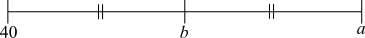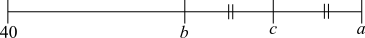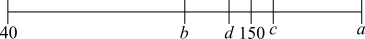Label the shortest distance $n$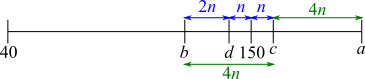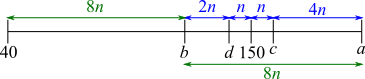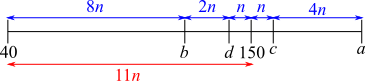$11n=$ distance from $40$ to $150$
$11n=110\Rightarrow n=10$
$a=150+n+4n=200$

Using weights
$b$ is made from $a$ and $40$, weighted equally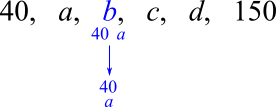$c$ is made from $a$ and ($a$ and $40$), but $a$ should have weight equal to the weight of ($a$ and $40$). That is the same as using $a$ twice and ($a$ and $40$) once.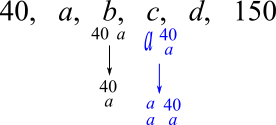$d$ is made from ($a$ and $40$) and (three $a$s and $40$), but again ($a$ and $40$) must be stretched to give equal weights: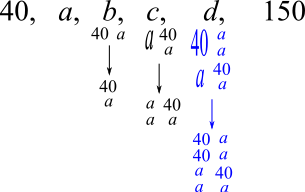And the same for $150$: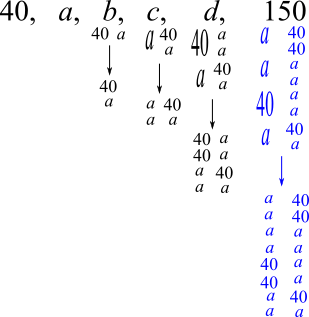$150$ is the average of eleven $a$s and five $40$s

$150=\dfrac {11a+200}{16}$

$\Rightarrow 16\times150 = 11a+200$
$\Rightarrow 2400 = 11a+200$
$\Rightarrow 2200 = 11a$ so $a=200$

Using algebra starting from the beginning (weights algebraically)
$\dfrac{40+a}2=b$ and $\dfrac{a+b}2 = c$

So $\dfrac{a+\frac{40+a}2}2=c \Rightarrow \dfrac{40+3a}4=c$

$d=\dfrac{b+c}2 = \dfrac{\frac{40+a}2+\frac{40+3a}4}2 = \dfrac{2(40+a)+40+3a}8 = \dfrac {120 + 5a} 8$

$150=\dfrac{c+d}2 = \dfrac{\frac{40+3a}4+\frac {120 + 5a} 8}2 = \dfrac{80+6a + 120+5a}{16} =\dfrac{200+11a}{16}$

$\Rightarrow 16\times150 = 200 + 11a$
$\Rightarrow 2400 = 200 + 11a$
$\Rightarrow 2200 = 11a$ so $a=200$

Using algebra starting from the end
$150$ is the mean of $c$ and $d$ so $c +d =300\Rightarrow d = 300 - c$ (make $d$ the subject to get rid of $d$, then get rid of $c$ etc until only $a$ remains)

$d$ is the mean of $b$ and $c$ so $b+c=2d=2(300-c)$
$\Rightarrow b+c=600-2c \Rightarrow 3c=600-b \Rightarrow c = \frac13(600-b)$

$c$ is the mean of $a$ and $b$ so $a+b=2c=2\times \frac13(600-b)$
$\Rightarrow 3a+3b=1200-2b \Rightarrow 5b = 1200-3a \Rightarrow b = \frac15(1200-3a)$

$b$ is the mean of $40$ and $a$ so $40+a=2b=2\times\frac15(1200-3a)$
$\Rightarrow 200+5a = 2400-6a \Rightarrow 11a = 2200 \Rightarrow a=200$

You can find more short problems, arranged by curriculum topic, in our short problems collection.